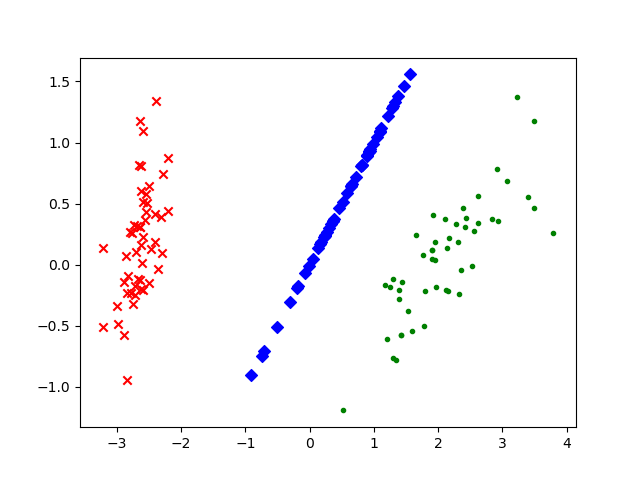# 主成分分析 PCA 算法

PCA是最常用的一种降维方法（非监督），可以用于提取数据的主要特征分量。

## 数学推导

PCA本质上是将方差最大的方向作为主要特征，并且在各个正交方向上将数据“离相关”，也就是让它们在不同正交方向上没有相关性。那么显然，推导PCA算法的关键就在于用方差定义样本间距，方差越大那么样本分布越稀疏。

$$\operatorname{Var}(a)=\frac{1}{m} \sum_{i=1}^{m} a_{i}^{2} \tag{2}$$

$$X=\left(\begin{array}{llll} a_{1} & a_{2} & \cdots & a_{m} \\ b_{1} & b_{2} & \cdots & b_{m} \end{array}\right)$$
$$\frac{1}{m} X X^{\top}=\left(\begin{array}{cc} \frac{1}{m} \sum_{i=1}^{m} a_{i}^{2} & \frac{1}{m} \sum_{i=1}^{m} a_{i} b_{i} \\ \frac{1}{m} \sum_{i=1}^{m} a_{i} b_{i} & \frac{1}{m} \sum_{i=1}^{m} b_{i}^{2} \end{array}\right)=\left(\begin{array}{ll} \operatorname{Cov}(a, a) & \operatorname{Cov}(a, b) \\ \operatorname{Cov}(b, a) & \operatorname{Cov}(b, b) \end{array}\right)$$

\begin{aligned} D &=\frac{1}{m} Y Y^{T} \\ &=\frac{1}{m}(P X)(P X)^{T} \\ &=\frac{1}{m} P X X^{T} P^{T} \\ &=P\left(\frac{1}{m} X X^{T}\right) P^{T} \\ &=P C P^{T} \end{aligned}

$$E^{T} C E=\Lambda=\left(\begin{array}{llll} \lambda_{1} & & & \\ & \lambda_{2} & & \\ & & \ddots & \\ & & & \lambda_{n} \end{array}\right)$$ 其中$\lambda$为各特征向量对应的特征值，因此可知需要的矩阵$P=E^T$

P 是协方差矩阵的特征向量单位化后按行排列出的矩阵，其中每一行都是 C 的一个特征向量。如果设 $P$ 按照 $\Lambda$​ 中特征值的从大到小，将特征向量从上到下排列，则用 $P$ 的前 $K$ 行组成的矩阵乘以原始数据矩阵 $X$，就得到了我们需要的降维后的数据矩阵 $Y$​。

## 代码实例

  1 2 3 4 5 6 7 8 9 10 11 12 13 14 15 16 17 18 19 20 21 22 23 24 25 26 27 28 29 30  import matplotlib.pyplot as plt from sklearn.decomposition import PCA from sklearn.datasets import load_iris data = load_iris() y = data.target x = data.data pca = PCA(n_components=2) reduced_x = pca.fit_transform(x) red_x, red_y = [], [] blue_x, blue_y = [], [] green_x, green_y = [], [] for i in range(len(reduced_x)): if y[i] == 0: red_x.append(reduced_x[i]) red_y.append(reduced_x[i]) elif y[i] == 1: blue_x.append(reduced_x[i]) blue_y.append(reduced_x[i]) else: green_x.append(reduced_x[i]) green_y.append(reduced_x[i]) plt.scatter(red_x,red_y,c='r',marker='x') plt.scatter(blue_x,blue_y,c='b',marker='D') plt.scatter(green_x,green_y,c='g',marker='.') plt.show()